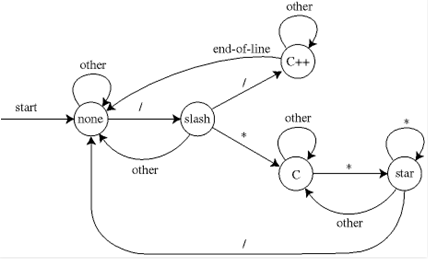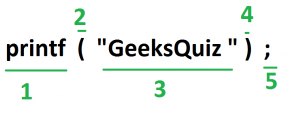Courses

# Lexical Analysis Computer Science Engineering (CSE) Notes | EduRev

## Computer Science Engineering (CSE): Lexical Analysis Computer Science Engineering (CSE) Notes | EduRev

The document Lexical Analysis Computer Science Engineering (CSE) Notes | EduRev is a part of the Computer Science Engineering (CSE) Course GATE Computer Science Engineering(CSE) 2022 Mock Test Series.
All you need of Computer Science Engineering (CSE) at this link: Computer Science Engineering (CSE)

Introduction

Lexical Analysis is the first phase of compiler also known as scanner. It converts the input program into a sequence of Tokens.

Lexical Analysis can be implemented with the Deterministic finite Automata.

What is a token?
A lexical token is a sequence of characters that can be treated as a unit in the grammar of the programming languages.

Example of tokens:

• Type token (id, number, real, . . . )
• Punctuation tokens (IF, void, return, . . . )
• Alphabetic tokens (keywords)
`Keywords; Examples-for, while, if etc.Identifier; Examples-Variable name, function name etc.Operators; Examples '+', '++', '-' etc.Separators; Examples ',' ';' etc`

Example of Non-Tokens:

• Comments, preprocessor directive, macros, blanks, tabs, newline  etc

How Lexical Analyzer functions
1. Tokenization .i.e Dividing the program into valid tokens.
2. Remove white space characters.
4. It also provides help in generating error message by providing row number and column number.The lexical analyzer identifies the error with the help of automation machine and the grammar of  the given language on which it is based like C , C++.

Suppose we pass a statement through lexical analyzer –

a = b + c ;                It will generate token sequence like this:

id=id+id;                 Where each id reference to it’s variable in the symbol table referencing all details

For example, consider the program

`int main(){  // 2 variables  int a, b;  a = 10; return 0;}`

All the valid tokens are:

`'int'  'main'  '('  ')'  '{'  '}'  'int'  'a'  'b'  ';' 'a'  '='  '10'  ';' 'return'  '0'  ';'  '}'`

Above are the valid tokens.
You can observe that we have omitted comments.

As another example, consider below printf statement.There are 5 valid token in this printf statement.
Exercise 1:
Count number of tokens :

`int main(){  int a = 10, b = 20;  printf("sum is :%d",a+b);  return 0;}Answer: Total number of token: 27.`

Exercise 2:

Count number of tokens :

int max(int i);

• Lexical analyzer first read int and finds it to be valid and accepts as token
• max is read by it and found to be valid function name after reading
• int  is also a token , then again i as another token and finally ;
`Answer:  Total number of tokens 7:     int, max, ( ,int, i, ), ;`

The document Lexical Analysis Computer Science Engineering (CSE) Notes | EduRev is a part of the Computer Science Engineering (CSE) Course GATE Computer Science Engineering(CSE) 2022 Mock Test Series.
All you need of Computer Science Engineering (CSE) at this link: Computer Science Engineering (CSE)Use Code STAYHOME200 and get INR 200 additional OFF Use Coupon Code
All Tests, Videos & Notes of Computer Science Engineering (CSE): Computer Science Engineering (CSE)

### Top Courses for Computer Science Engineering (CSE)## GATE Computer Science Engineering(CSE) 2022 Mock Test Series

131 docs|159 tests

### Top Courses for Computer Science Engineering (CSE)Track your progress, build streaks, highlight & save important lessons and more!

,

,

,

,

,

,

,

,

,

,

,

,

,

,

,

,

,

,

,

,

,

;